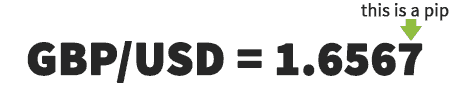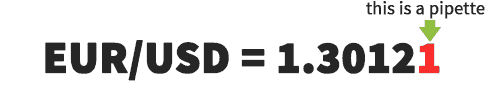# What is a Pip in Forex?

What is a Pip? A “pip” is a unit of measurement used to show changes in the rate of a pair. In the image below, a pip is the fourth decimal.Pips are one of the ways by which traders calculate how much profit they made or lost on a trade. For example, if you enter a long position on GBP/USD at 1.6550 and it moves to 1.6600 by the time you close your position you have made a 50 pip profit.

If you enter a short position at 1.6550 and the price moves up to 1.6600 you lose 50 pips. Remember, short means you want the rate to go down. So, if you short at 1.6550 and price falls to 1.6500, you make 50 pips profit. Below are a few more examples of trades.

Long entry at 172.50
Exit at 172.87
Profit/loss = +37 pips

Long entry at 1.6077
Exit at 1.6007
Profit/Loss = -70 pips

Short entry at 1.3491
Exit at 1.3191
Profit/Loss = +300 pips

Short entry at 1.0863
Exit at 1.0830
Profit/Loss = +33 pips

Long entry at 1.4058
Exit at 1.3058
Profit/Loss = -1000 pips

You may have noticed that in all the examples above, the pip is either in the fourth or second decimal place. For example, on EUR/USD the pip is the fourth decimal, on GBP/JPY the pip is the second decimal. The fourth and second decimal place are the standard in Forex. Virtually every pair you trade will have the pip as either the fourth or second decimal.

Now that you know what a pip is, and how to calculate pip gains and losses, you may be asking yourself “how much money is each pip worth?”

## How Much is a Pip Worth?

The value of a pip changes depending on the pair you trade. Calculating the value of a pip is not vital to your success, as a trader, since your broker will automatically calculate the value for you. However, if you’re going to trade, you should know a little about how this works.

On GBP/USD a pip is the fourth decimal place, 0.0001. So, if you enter long at 1.6400 and the rate of GBP/USD moves up to 1.6450, you have made 50 pips, or 0.0050.

Calculating the current value of a pip is easy. Here is the simple formula:

1 pip / exchange rate = value per pip

Lets take a look at a few examples.

USD/CHF = 1.0810

0.0001 / 1.0810 = 0.00009250
1 PIP = 0.00009250 USD

Clearly, this is not much money. However, through leverage, \$0.00009250 can become a significant amount. You will learn about leverage later.

USD/JPY = 96.27

0.01 / 96.27= 0.0001038
1 PIP = 0.0001038 USD

USD/CHF = 1.0810

0.0001 / 1.0810 = 0.00009250
1 PIP = 0.00009250 USD

In the next two examples, because the base currency is not USD, using the same equation as above we get the value of a pip in the base currency. So for GBP/USD the pip value will be shown in GBP not USD.

However, if you trade in USD you may want the pip value in USD. On any pair, with USD as the quote currency, to get the pip value in USD you simply multiply the pip value by the exchange rate:

pip value x exchange rate = pip value in USD

GBP/USD = 1.6443

0.0001/ 1.6443= 0.00006081
1 PIP = 0.00006081 GBP

0.00006081 x 1.6443 = 0.00009998 USD

This is rounded up to 0.0001 USD

EUR/USD = 1.3940

0.0001/ 1.3940= 0.00007173
1 PIP = 0.00007173 EUR

0.00007173 x 1.3940= 0.00009999 USD

This is rounded up to 0.0001 USD

You will find that all pairs with USD as the quote currency have a pip value of roughly \$0.00001 USD.

The next (and thankfully the last) example shows how to calculate the value of a pip, in USD, for pairs that do not have USD as either the base or quote pair.

GBP/JPY = 158.80

0.01/ 158.80= 0.00006297
1 PIP = 0.00006297 GBP

This time because the quote currency is JPY, multiplying by the exchange rate will give you the pip value in JPY. So to get this to USD you simply take the GBP/USD rate and multiply the pip value by it.

0.00006297 x 1.6443 = 0.0001035
1 PIP = 0.0001035 USD

How boring was that? As is said above it is not vital to know this stuff. However, if you’re going to trade Forex, you might as well know how it all works.

## So, what about a pipette?

In the last few years, some Forex brokers have started displaying an additional decimal at the end of a currency pairs rate.This additional decimal is know as a pipette, or a micro pip. A pipette is simply one tenth of a pip. The pipette will appear as either the fifth or third decimal place in a currency pairs rate.

If your broker displays pipettes, do not worry. You can simply ignore the pipette when you are calculating how many pips you have made or lost on a trade.

Now, next time somebody asks you “What is a pip?” or “What is a pipette?”, you can explain it to them. Next I will teach about lots, leverage, and margin.